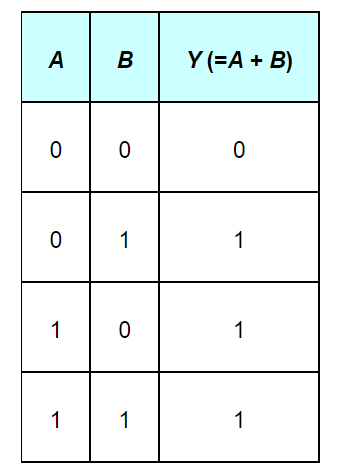# Write the truth table for circuit given in Fig.`
Question:

Write the truth table for circuit given in Fig. 14.47 below consisting of NOR gates and identify the logic operation (OR, AND, NOT) which this circuit is performing.(Hint: A = 0, B = 1 then A and B inputs of second NOR gate will be 0 and hence Y=1. Similarly work out the values of Y for other combinations of A and B. Compare with the truth table of OR, AND, NOT gates and find the correct one.)

Solution:

$A$ and $B$ are the inputs of the given circuit. The output of the first NOR gate is $\overline{A+B}$. It can be observed from the following figure that the inputs of the second NOR gate become the out put of the first one.Hence, the output of the combination is given as:The truth table for this operation is given as:This is the truth table of an OR gate. Hence, this circuit functions as an OR gate.